# Geometry basic rules

Martin McBride, 2020-08-15
Tags angles in triangle angles in quadrilateral exterior angle angles on point angles around line isosceles triangle
Categories maths gcse geometry

Here are some basic geometric rules that are useful to know. They are also covered in this video:

## Angles around a point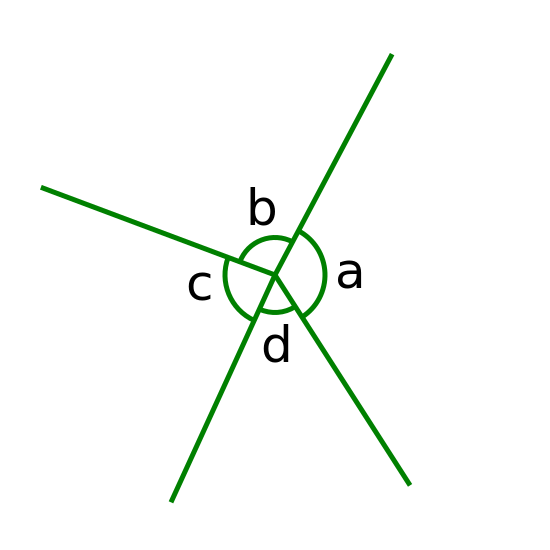The angles around a point add up to 360°.

a + b + c + d = 360°


## Angles on a line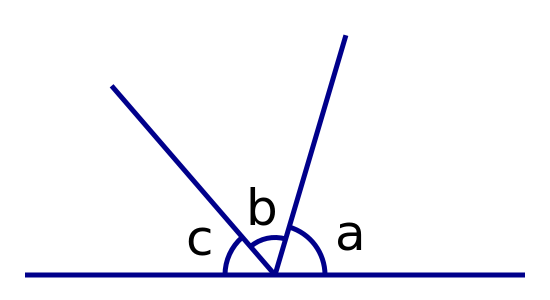The angles on a straight line add up to 180°.

a + b + c = 180°


## Angles in a triangle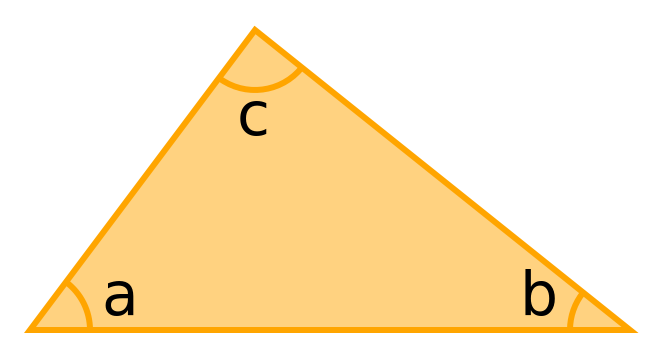The interior angles in a triangle add up to 180°.

a + b + c = 180°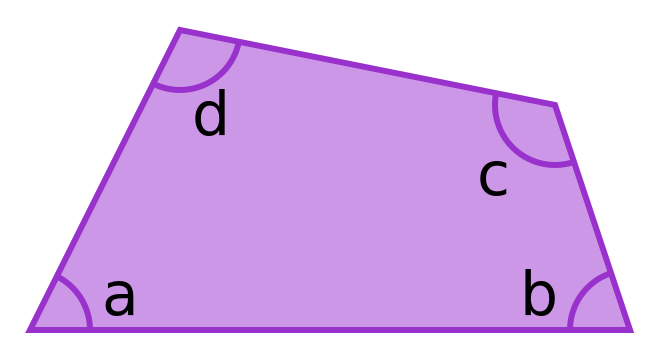The interior angles in a quadrilateral (any 4-sided shape) add up to 360°.

a + b + c + d = 360°


## Exterior angles of a triangle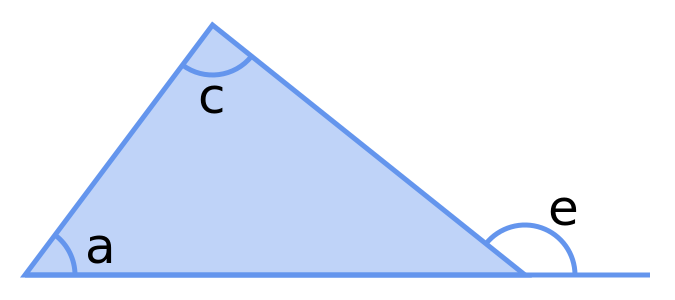An exterior angle of a triangle is equal to the sum of the 2 opposite interior angles.

e = a + b


## Angles and sides of an isosceles triangle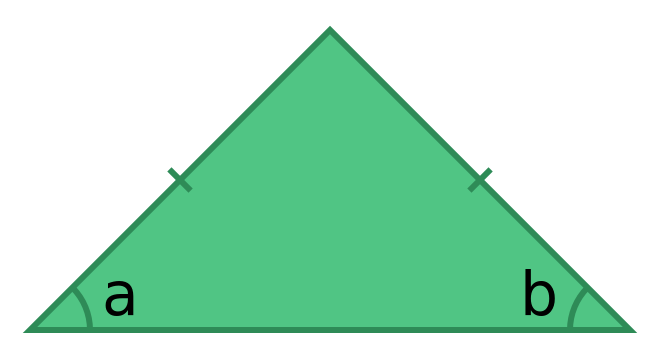An isosceles triangle has two sides of equal length (the two sides indicated by the tick marks that are drawn across the lines). It also has two equakl angles:

a = b